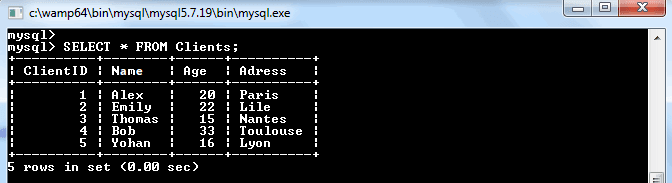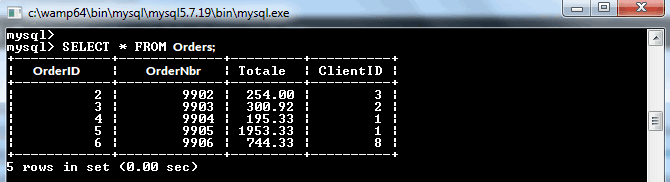# MySQL Derived Table

If your interested to know about the export table to CSV file in MySQL

A derived table in MySQL is a virtual table that returned from the SELECT…FROM statement. In other words, it is an expression, which generates a table under the scope of the FROM clause in the SELECT statement. This concept is similar to the temporary table. However, the derived table is simpler than a temporary table because there is no need to use all steps that temporary tables need for its creation.

Most developers used the term derived table and subquery interchangeably. Thus, we can say that when we use a subquery in the SELECT…FROM clause, it is called a derived table.

The following image illustrates a query that uses a derived table:

### Syntax

The following are the syntax to use the derived table in MySQL:

`SELECT ... FROM (subquery) [AS] table_name (column_list) WHERE condition;  `

It is to note that the stand-alone subquery is a subquery, which can be executed independently of the statement that contains this query. e should also consider that a derived table must contain the alias table name that allows us to reference its name later in the statement. If we do not provide the alias name to a derived table, MySQL will issue the below error message:

Every derived table must have its own alias.

It is also mandatory that each column of the derived table should have unique names. Let us understand it with the help of the following illustration. First, we will create a table using the below statement:

`mysql> CREATE TABLE test1 (s1 INT, s2 CHAR(5), s3 FLOAT);  `

Next, fill some record into this table using the below statement:

`mysql> INSERT INTO test1 VALUES (1, 'A', 10.0), (2, 'B', 20.0), (3, 'C', 30.0);   `

Execute the SELECT statementto verify the output:

Now, execute the below statement to understand how we can use a subquery in the FROM clause.

```SELECT sb1, sb2, sb3
FROM (SELECT s1 AS sb1, s2 AS sb2, s3*5 AS sb3 FROM test1) AS test1_set
WHERE sb1 < 4;  ```

It will return the output as follows:

Let us another example where we will get the average of the float value column. Execute the below statement:

```SELECT AVG(sum_float)
FROM (SELECT SUM(s3) AS sum_float FROM test1 GROUP BY s1) AS totals;  ```

In the above statement, the derived table calculates the total sum of the float column and then returns the average from the outer query. See the below output:

### Derived tables in MySQL have the following restrictions:

• MySQL does not use a correlated subquery as a derived table.
• MySQL does not allow a derived table to use references to other tables of the same SELECT statement.
• We cannot use outer references in the derived table.
• A derived table cannot contain references to other tables of the same `SELECT`  a derived table cannot contain outer references. This is a MySQL restriction that is lifted in MySQL 8.0.14, not a restriction of the SQL standard. For example, the derived table `dt` in the following query contains a reference `t1.b` to the table `t1` in the outer query:
```<code>SELECT * FROM t1 WHERE t1.d > (SELECT AVG(dt.a) FROM (SELECT SUM(t2.a)
AS a FROM t2 WHERE t2.b = t1.b GROUP BY t2.c)
dt WHERE dt.a > 10);</code>The query is valid in MySQL 8.0.14 and higher.
Before 8.0.14, it produces an error: <code>Unknown column 't1.b' in 'where clause'</code>```

## Example:

In the example below, we have two tables, called “Clients” and “Orders”, which contain the following data:Now the following query will produce a derived table:SelectAVG(T) FROM( SELECT OrderNbr, SUM(Total) TFROM Orders GROUPBY OrderNbr)AS totals;

Output:

```+----------------+
|     AVG(T)     |
+----------------+
|     689.582000 |
+----------------+```

We must provide an alias for all derived tables. In this case, we have given our derived table the alias “totals”. If we run only the subquery, we can see the result of the derived table.SELECT OrderNbr, SUM(Total) TFROM OrdersGROUPBY OrderNbr;

Output:

```+----------+-----------+
| OrderNbr |     T     |
+----------+-----------+
|   9902   |  254.00   |
|   9902   |  300.92   |
|   9902   |  195.33   |
|   9902   |  1953.33  |
|   9902   |  744.33   |
+----------+-----------+```

## A simple MySQL derived table example

The following query gets the top five products by sales revenue in 2003 from the `orders` and `orderdetails` tables in the sample database:

```<code>SELECT
productCode,
ROUND(SUM(quantityOrdered * priceEach)) sales
FROM
orderdetails
INNER JOIN
orders USING (orderNumber)
WHERE
YEAR(shippedDate) = 2003
GROUP BY productCode
ORDER BY sales DESC
LIMIT 5;</code><small>Code language: SQL (Structured Query Language) (sql)</small>```
MySQL Derived Table